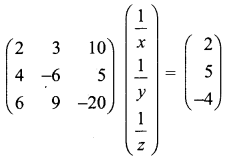You can Download Samacheer Kalvi 12th Maths Book Solutions Guide Pdf, Tamilnadu State Board help you to revise the complete Syllabus and score more marks in your examinations.

## Tamilnadu Samacheer Kalvi 12th Maths Solutions Chapter 1 Applications of Matrices and Determinants Ex 1.3

Question 1.
Solve the following system of linear equations by matrix inversion method:
(i) 2x + 5y = -2, x + 2y = -3
(ii) 2x – y = 8, 3x + 2y = -2
(iii) 2x + 3y – z = 9, x + y + z = 9, 3x – y – z = -1
(iv) x + y + z – 2 = 0, 6x – 4y + 5z – 31 = 0, 5x + 2y + 2z = 13
Solution:
(i) 2x + 5y = -2, x + 2y = -3
The matrix form of the above equations is $$\left(\begin{array}{ll}{2} & {5} \\ {1} & {2}\end{array}\right)\left(\begin{array}{l}{x} \\ {y}\end{array}\right)=\left(\begin{array}{l}{-2} \\ {-3}\end{array}\right)$$
(i.e) AX = B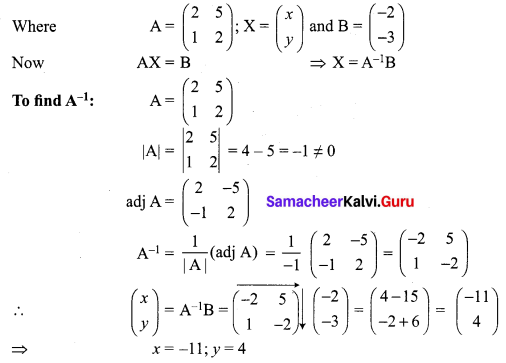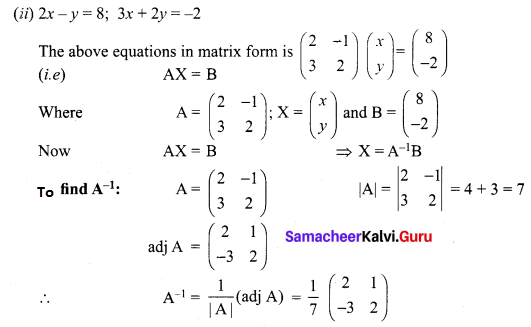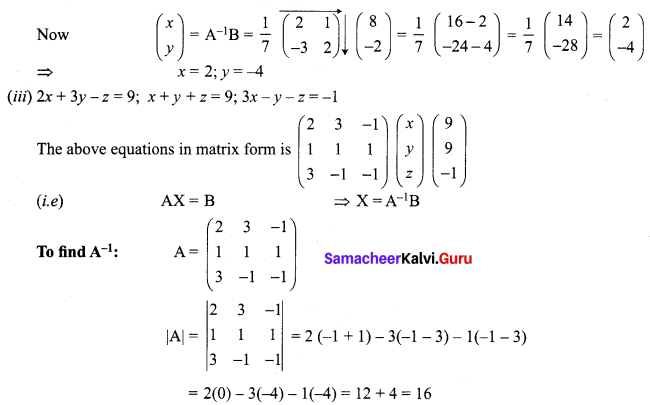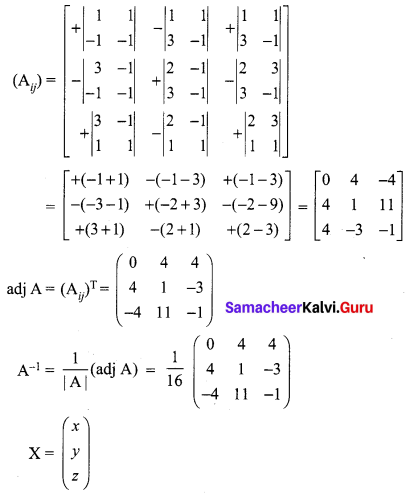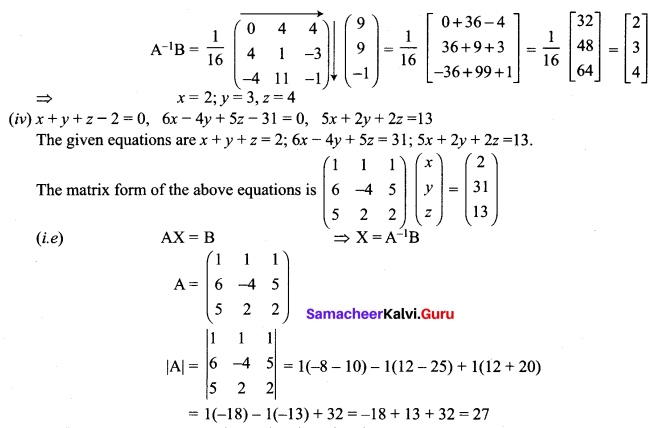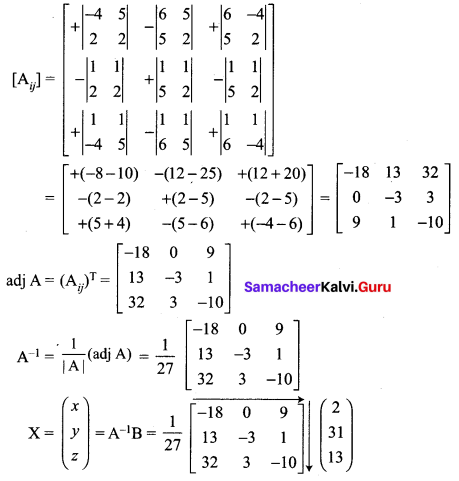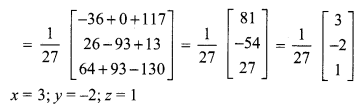Question 2.
If A = $$\left[\begin{array}{ccc}{-5} & {1} & {3} \\ {7} & {1} & {-5} \\ {1} & {-1} & {1}\end{array}\right]$$ and B = $$\left[\begin{array}{lll}{1} & {1} & {2} \\ {3} & {2} & {1} \\ {2} & {1} & {3}\end{array}\right]$$ find the products AB and BA and hence solve the system of equations x + y + 2z = 1, 3x + 2y + z = 7, 2x + y + 3z = 2.
Solution: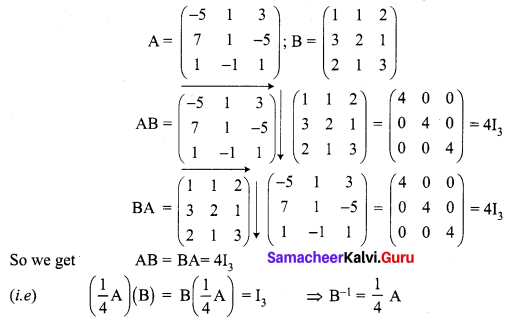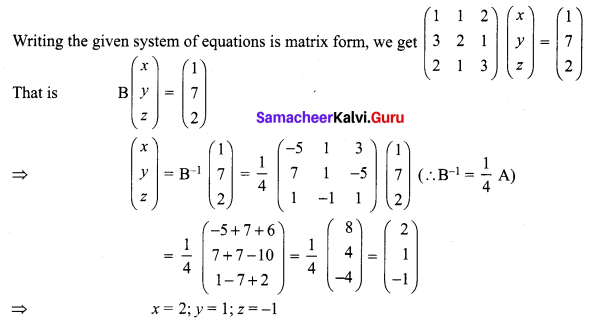Question 3.
A man is appointed in a job with a monthly salary of certain amount and a fixed amount of annual increment. If his salary was ₹ 19,800 per month at the end of the first month after 3 years of service and ₹ 23,400 per month at the end of the first month after 9 years of service, find his starting salary and his annual increment. (Use matrix inversion method to solve the problem.)
Solution:
Let his monthly salary be ₹ x and his annual increment be ₹ y
Given x + 3y = 19800 and x + 9y = 23400
Writing the above equations in matrix form, we get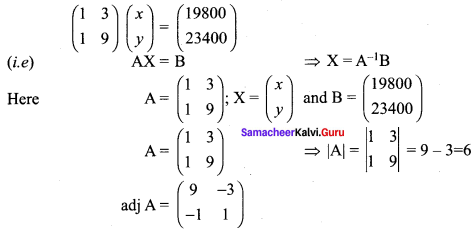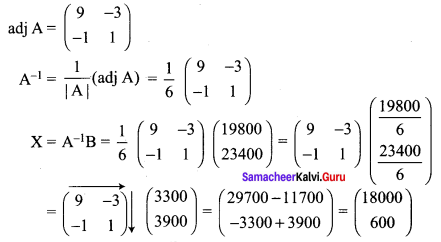x = ₹ 18000 and y = ₹ 600
(i.e) monthly salary = ₹ 18000 and annual increment = ₹ 600

Question 4.
4 men and 4 women can finish a piece of work jointly in 3 days while 2 men and 5 women can finish the same work jointly in 4 days. Find the time taken by one man alone and that of one woman alone to finish the same work by using matrix inversion method.
Solution:
Let the work done by man in 1 day be x and the work done by a woman in 1 day be y
Now we are given
4x + 4y = $$\frac { 1 }{ 3 }$$ ⇒ 12x + 12y = 1
and 2x + 5y = $$\frac { 1 }{ 4 }$$ ⇒ 8x + 20y = 1
The matrix form of the above equations is $$\left(\begin{array}{cc}{12} & {12} \\ {8} & {20}\end{array}\right)\left(\begin{array}{l}{x} \\ {y}\end{array}\right)=\left(\begin{array}{l}{1} \\ {1}\end{array}\right)$$
(i.e) AX = B ⇒ X = A-1B
Here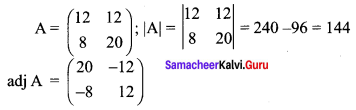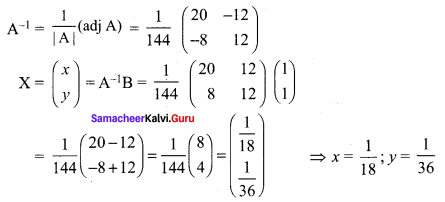(i.e) The work done by a man in 1 day = x = $$\frac{1}{18}$$
The time taken by a man to finish the work = 18 days.
The work done by a woman in 1 day = y = $$\frac{1}{36}$$
The time is taken by a woman to finish the work = 36 days.

Question 5.
The prices of three commodities A, B and C are ₹ x, y and z per units respectively. A person P purchases 4 units of B and sells two units of A and 5 units of C. Person Q purchases 2 units of C and sells 3 units of A and one unit of B . Person R purchases one unit of A and sells 3 unit of B and one unit of C. In the process, P, Q and R earn ₹ 15,000, ₹ 1,000 and ₹ 4,000 respectively. Find the prices per unit of A,B and C. (Use matrix inversion method to solve the problem.)
Solution:
Price of A = ₹ x /unit
Price of B = ₹ y /unit
Price of C = ₹ z /unit
We are given
2x – 4y + 5z = 15000
3x + y – 2z = 1000
-x + 3y + z = 4000
The matrix form of the above equations is $$\left(\begin{array}{rrr}{2} & {-4} & {5} \\ {3} & {1} & {-2} \\ {-1} & {3} & {1}\end{array}\right)\left(\begin{array}{l}{x} \\ {y} \\ {z}\end{array}\right)=\left(\begin{array}{r}{15000} \\ {1000} \\ {4000}\end{array}\right)$$
(i.e) AX = B ⇒ X = A-1B
Here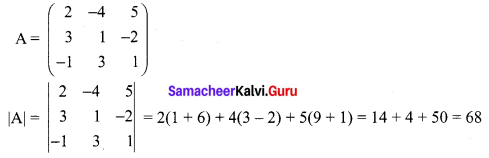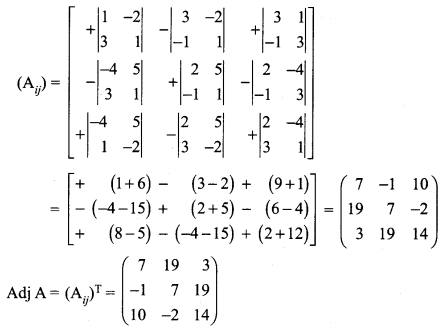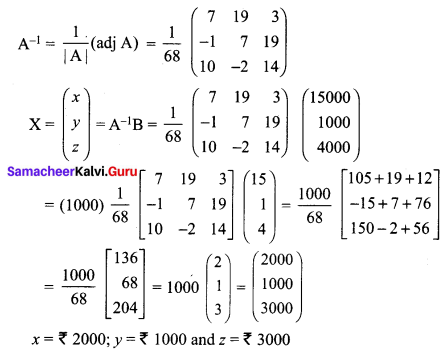### Samacheer Kalvi 12th Maths Solutions Chapter 1 Applications of Matrices and Determinants Ex 1.3 Additional Problems

Question 1.
Using matrix method, solve the following system of equations:
x + 2y + z = 7, x + 3z = 11, 2x – 3y = 1
Solution:
The system of equations can be written in the form AX = B, where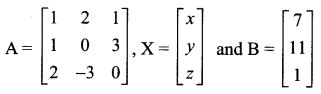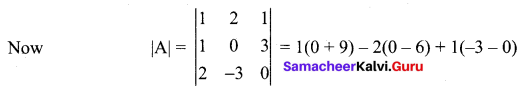= 9 + 12 – 3 = 18 ≠ 0 Non-singular matrix.
A11 = (0 + 9) = 9, A12 = -(0 – 6) = 6,
A13 = (-3 – 0) = -3
A21 = – (0 + 3) = -3, A22 = (0 – 2) = -2
A23 = -(-3 – 4) = 7
A31 = (6 – 0) = 6, A32 = -(-3 – 1) = -2,
A33 = (0 – 2) = -2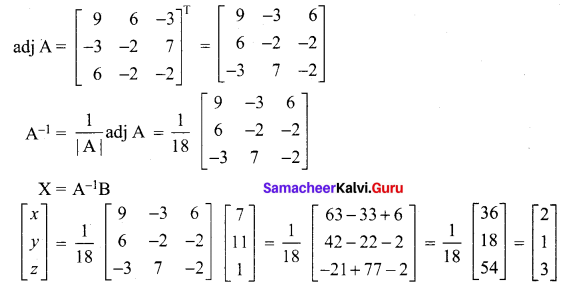Thus x = 2 ,y = 1 and z = 3.

Question 2.
Using matrices, solve the following system of linear equations: x + 2y – 3z = -4, 2x + 3y + 2z = 2, 3x – 3y – 4z = 11.
Solution:
The system of equations can be written in the form AX = B, where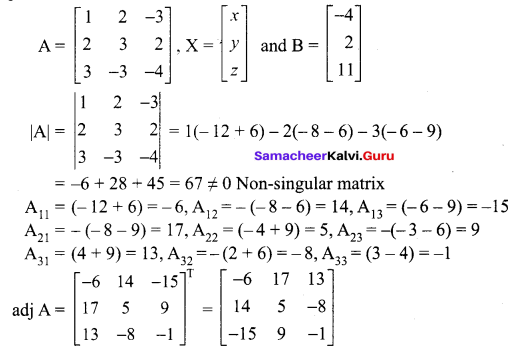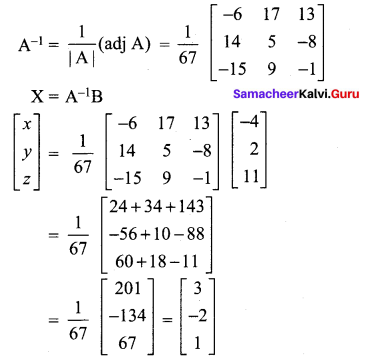Thus x = 3,
y = -2 and z = 1Question 3.
If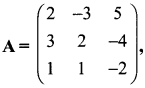Find A-1. Hence using A-1 solve the system of equations.
2x – 3y + 5z = 11,
3x + 2y – 4z = -5,
x + y – 2z = -3.
Solution: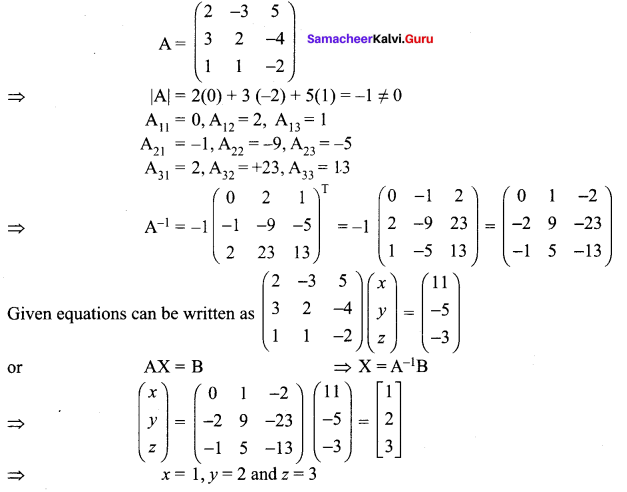Question 4.
Use product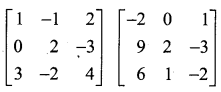to solve the system of equations
x + 3z = 9,
-x + 2y – 2z = 4,
2x – 3y + 4z = -3
Solution: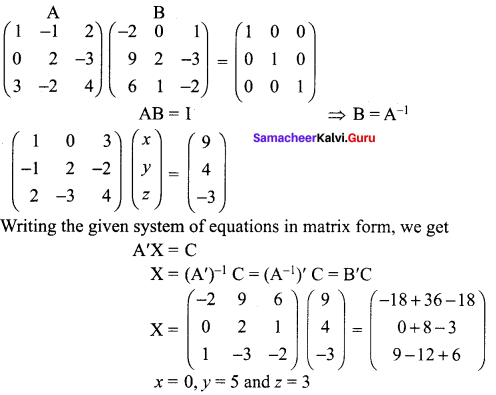Question 5.
Use product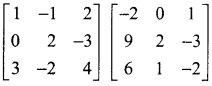to solve the system of equations
x – y + 2z = 1,
2y – 3z = 1,
3x – 2y + 4z = 2
Solution: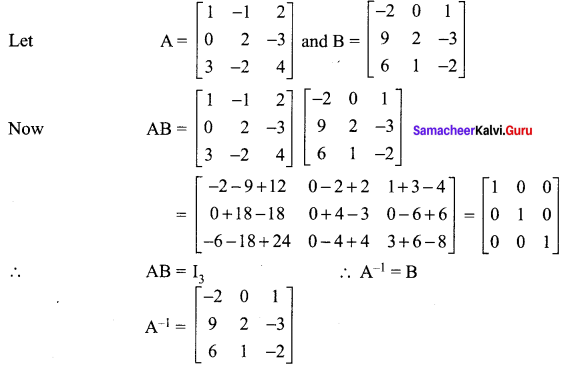The system of equations can be written in the form AX = C, where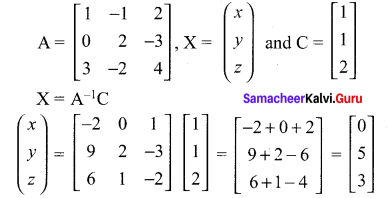Question 6.
An amount of ₹ 7000 is invested in three types of investments x, y and z at the rate of 3%, 4% and 5% interest respectively. The total annual income is ₹ 280. If the combined income from x and y is ₹ 80 more than that from z, then
(i) Represent the above situation in form of linear equations .
(ii) Is it possible to frame the given linear equations in the form of matrix to obtain the three values x, y and z using matrix multiplication? If yes, find.
(iii) Which value is more beneficial to invest?
Solution: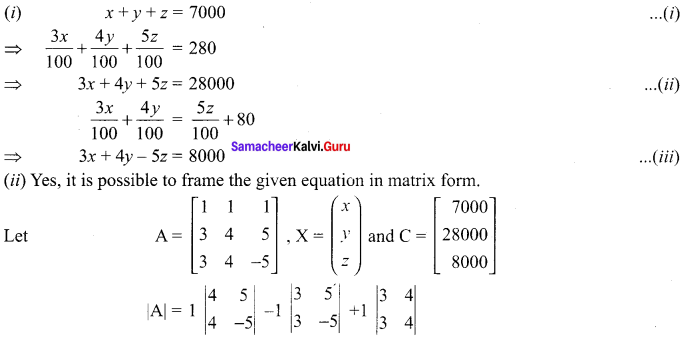⇒ -40 + 30 + 0 = -10 ≠ 0
Cofactors C11 = +(-20 – 20) = -40
C21 = -(5 – 4) = 9
C31 = +(5 – 4) = 1
C12 = +(-15 – 15) = -30
C22 = +(-5 – 3) = -8
C32 = -(5 – 3) = -2
C13 = +(12 – 12) = 0
C23 = -(4 – 3) = -1
C33 = +(4 – 3) = 1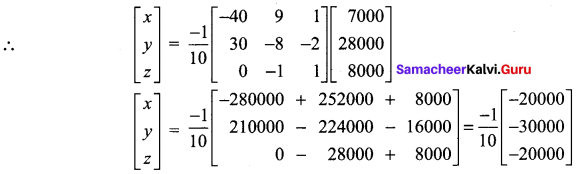Thus, the three values x = ₹ 2000, y = ₹ 3000 and z = ₹ 2000
(iii) The value of y is more beneficial to invest

Question 7.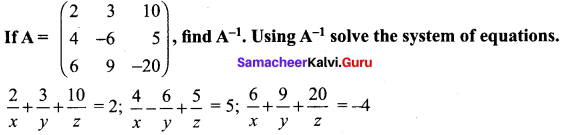Solution: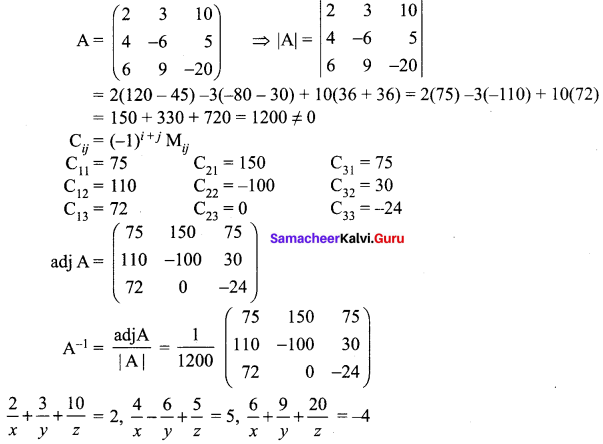In matrix form